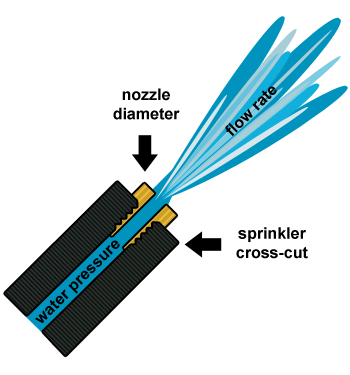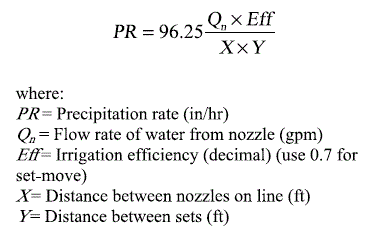# Nozzle Flow Rate and Effective Application Rate

Use this first form to determine the effective application rate of sprinklers spaced at uniform distances from each other. This is particularly applicable to hand-move, or wheel-line, irrigation systems. The pressure is measured at the sprinkler nozzle. The head spacing is the distance between sprinkler heads along the water line, and the line spacing is the distance between lines in the field. If there is just one line being moved and the spray patterns overlay, as is typical for hand-move or wheel-line, then the actual application rate will be lower but the given number will be useful to determine set times.

## Nozzle Flow Rate and Effective Application Rate

Nozzle Diameter:
Pressure:
Line Spacing:
Sprinkler Efficiency:
(Minimum efficiency of DuCaR Sprinklers is 80%)
%
Nozzle Flow Rate:
Effective Application Rate:

### The Equation

This Calculator uses these equations to determine the flow rate, nozzle diameter and effective application rate.Reference: Washington State University